# Javascript String Slice Method

Javascript String Slice Method

As the name of the method says, we are going to use this method to slice the given string, which means that we will just get some portion of the string, as another string. This is pretty easy to be implemented, and below, we are going to see the implementation of the slice method.

The question, for now, is, what arguments should be passed on to the slice method, so that we get the appropriate output? Well, the thing is that the slice method requires two things, the starting index, and the ending index(remember that the ending index is not included). So, this is like we are going to get the portion of the string, from the specified starting index to the specified ending index(not included).

The slice method is going to return a string, with contents from the start position, to the end position (the end is not included). So, this is like we can slice some string from index 3 to index 8, and we will get the string contents from index 3 to index 7 because 8 is not considered.

Now, let’s have a look at a simple program, which demonstrates the slice method easily.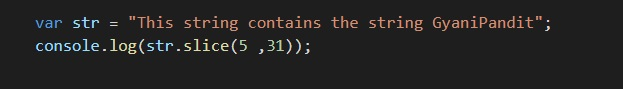In the above program, there is a string, with the variable name str, and on that string, we are applying the slice method here. The method is given the start position as 5 and the end position as 31, so the string would be sliced accordingly, and would be returned. We are not storing it anywhere, and just putting it onto the console.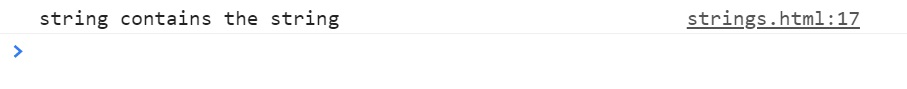You can also try giving some negative arguments to the method slice. If everything is alright, the method will return the string, but this time, from the last. Let’s have a look at this as well –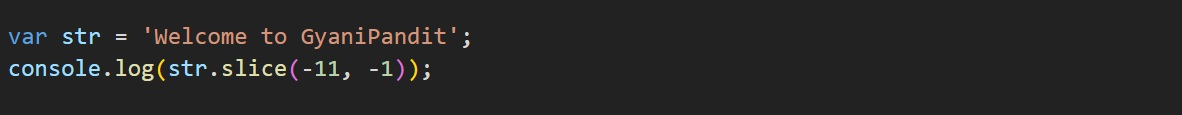As you can see, the starting index this time given is -11, and the ending index is -1. So, this time, the output that we get is something like this –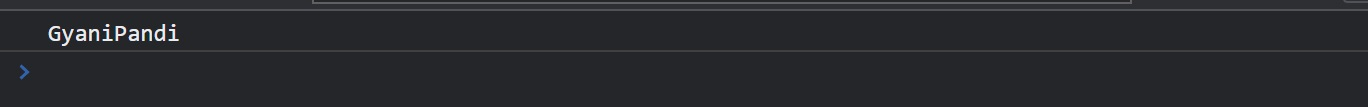As you can see, we got the output from the starting index -11, to the ending index -2, since -1 would not be included. Also, if the arguments that you are passing are not valid, for example, if the starting position is found to be greater than the ending position, then the slice method is going to return an empty string here. You can try doing this, and observe the output as well.

Also, another thing to note is that if we are providing only the starting index and not the ending index, then what is going to happen? Will it give an error, because we did not give all the things that it requires?

Well, this is not the case here. If we are giving only one argument, it will be the starting index, and from the starting index to the end of the string, the string will be sliced. You can try doing this thing as well, but let’s consider an example over here, so you can get the idea.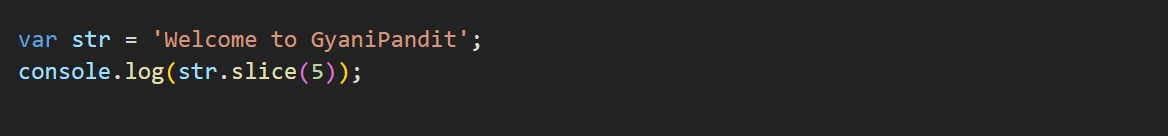As you can see, we have given only one argument to the slice method, and that is the starting position to slice. The ending position is not specified, so it would consider the end of the string. So, the string that we are going to get here, would contain all the things from the index 5 to the end of the string. Let’s have a look at the output now –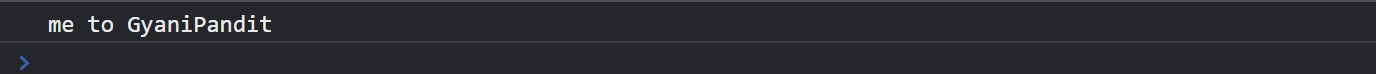As you can see, the string now starts from the index 5(from ‘m’), and goes to the end of the string, since the end position is not specified. You can try the slice method in different terms, and we saw some examples over here, to get a clear idea about the method.